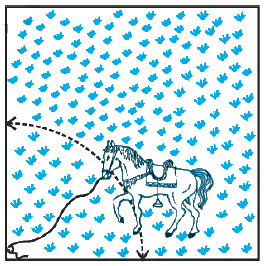# Ex.12.2 Q8 Areas Related to Circles Solution - NCERT Maths Class 10

## Question

A horse is tied to a peg at one corner of a square shaped grass field of side $$15\,\rm{m}$$ by means of a $$5 \,\rm{m}$$ long rope (see given figure). Find

(i) the area of that part of the field in which the horse can graze.

(ii) the increase in the grazing area if the rope were $$10 \,\rm{m}$$ long instead of $$5\, \rm{m.}$$

(Use $$\pi = 3.14$$)

Video Solution
Areas Related To Circles
Ex 12.2 | Question 8

## Text Solution

What is known?

Length of the side of the square grass field  $$= 15 \,\rm{m}$$ and rope of length $$= 5\,\rm{m}$$  by which a horse is tied to a peg at one corner of the field.

What is unknown?

(i) Area of the field the horse can graze

(ii) Increase in grazing area if the rope were $$10\,\rm {m}$$ long instead of $$5 \,\rm{m}$$

Reasoning:

(i) From the figure it’s clear that the horse can graze area of a a sector of a circle with radius$$\rm{}(r)$$ $$\rm{}5\,m$$and angle with degree measure $${90^o}$$ (as the peg is at corner of a square and angle of square $$= {90^o}$$) length of the rope  $$= 5\,\rm{m}$$

$$\therefore \quad$$Area of a horse can graze $$=$$ Area of a sector

\begin{align}&= \frac{\theta }{{{{360}^\circ }}} \times \pi {r^2}\\&= \frac{{{{90}^\circ }}}{{{{360}^\circ }}} \times \pi {r^2}\\&= \frac{{\pi {r^2}}}{4}\end{align}

(ii) Similar to the first part

Area that can be grazed by the horse when length of the rope is $$\rm{}10\,m$$ is area of a sector of a circle with radius(r1) 10m and angle with degree measure $$\rm{}90^\circ$$

Area of the field horse can graze = Area of the sector

\begin{align} &= \frac{{{{90}^\circ }}}{{{{360}^\circ }}} \times \pi r_1^2 \\&= \frac{{\pi {r_1}^2}}{4} \end{align}

Increase in grazing area

\begin{align}& = \frac{{\pi r_1^2}}{4} - \frac{{\pi {r^2}}}{4}\\ &= \frac{\pi }{4}\left( {r_1^2 - {r^2}} \right)\end{align}

Steps:(i) Area of the field the horse can graze = Area of sector of \begin{align}90^{\circ}\end{align} in a circle of radius $$5\,\rm{m}$$

\begin{align} &= {\frac{{{{90}^\circ }}}{{{{360}^\circ }}} \times \pi {{(5)}^2}}\\&={ \frac{{25}}{4} \times 3.14}\\&= \frac{{78.5}}{4}\\& = 19.625\,\rm{{{m}}^2}\end{align}

(ii) Area that can be grazed by the horse when rope is $$10\,\rm{m}$$ long.

\begin{align} &={\frac{{{{90}^\circ }}}{{{{360}^\circ }}} \times \pi {{(10)}^2}}\\&= {\frac{1}{4} \times 100 \times 3.14}\\&= \frac{{314}}{4}\\& = 78.5\,\rm{{{m}}^2}\end{align}

Increase in grazing area$$= 78.5{\rm{}m^2} - 19.625\,{\rm{}m^2} = 58.875{\rm{}m^2}$$

$$\therefore \quad$$Increase in grazing area \begin{align} &=(78.5-19.525)\, \rm{m}^{2} \\ &=58.875\, \rm{m}^{2} \end{align}

Learn from the best math teachers and top your exams

• Live one on one classroom and doubt clearing
• Practice worksheets in and after class for conceptual clarity
• Personalized curriculum to keep up with school